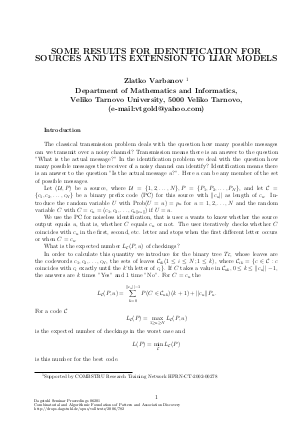Document# Some Results for Identification for Sources and its Extension to Liar Models

### Author Zlatko Varbanov## File

DagSemProc.06201.8.pdf
• Filesize: 126 kB
• 4 pages

## Cite As

Zlatko Varbanov. Some Results for Identification for Sources and its Extension to Liar Models. In Combinatorial and Algorithmic Foundations of Pattern and Association Discovery. Dagstuhl Seminar Proceedings, Volume 6201, pp. 1-4, Schloss Dagstuhl - Leibniz-Zentrum für Informatik (2006)
https://doi.org/10.4230/DagSemProc.06201.8

## Abstract

Let (\${cal U}, P\$) be a source, where \${cal U} = {1,2,dots,N}, P = {P_1, P_2, dots, P_N}\$, and let \${cal C} = {c_1,c_2,dots,c_N}\$ be a binary prefix code (PC) for this source with \$||c_u||\$ as length of \$c_u\$. Introduce the random variable \$U\$ with Prob(\$U=u\$) = \$p_u\$ for \$u = 1,2,dots,N\$ and the random variable \$C\$ with \$C = c_u = (c_1,c_2,dots,c_{u||c_u||})\$ if \$U=u\$. We use the PC for noiseless identification, that is user \$u\$ wants to know whether the source output equals \$u\$, that is, whether \$C\$ equals \$c_u\$ or not. The user iteratively checks whether \$C\$ coincides with \$c_u\$ in the first, second, etc. letter and stops when the first different letter occurs or when \$C = c_u\$. What is the expected number \$L_{cal C}(P,u)\$ of checkings? In order to calculate this quantity we introduce for the binary tree \$T_{cal C}\$, whose leaves are the codewords \$c_1,c_2,dots,c_N\$, the sets of leaves \${cal C}_{ik} (1 leq i leq N; 1 leq k)\$, where \${cal C}_{ik} = {c in {cal C}: c\$ coincides with \$c_i\$ exactly until the \$k\$'th letter of \$c_i}\$. If \$C\$ takes a value in \${cal C}_{uk}, 0 leq k leq ||c_u||-1\$, the answers are \$k\$ times "Yes" and 1 time "No". For \$C = c_u\$ the \$\$ L_{cal C}(P,u) = sum_{k=0}^{||c_u||-1}P(C in {cal C}_{uk})(k+1) + ||c_u||P_u. \$\$ For code \${cal C}\$,~ \$L_{cal C}(P) = max L_{cal C}(P,u)\$, \$1 geq u geq N\$, is the expected number of checkings in the worst case and \$L(P) = min L_{cal C}(P)\$ is this number for the best code \${cal C}\$. Let \$P = P^N = {frac{1}{N}, dots, frac{1}{N}}\$. We construct a prefix code \${cal C}\$ in the following way. In each node (starting at the root) we split the number of remaining codewords in proportion as close as possible to \$(frac{1}{2},frac{1}{2})\$. It is known that \$\$ lim_{N ightarrow infty} L_{cal C}(P^N) = 2 \$\$ (Ahlswede, Balkenhol, Kleinewachter, 2003) We know that \$L(P) leq 3\$ for all \$P\$ (Ahlswede, Balkenhol, Kleinewachter, 2003). Also, the problem to estimate an universal constant \$A = sup L(P)\$ for general \$P = (P_1,dots, P_N)\$ was stated (Ahlswede, 2004). We compute this constant for uniform distribution and this code \${cal C}\$. \$\$ sup_N L_{cal C}(P^N) = 2+frac{log_2(N-1)-2}{N} \$\$ Also, we consider the average number of checkings, if code \${cal C}\$ is used: \$ L_{cal C}(P,P) = sum P_u L_{cal C}(P,u)\$, for \${u in {cal U}}\$. We calculate the exact values of \$L_{cal C}(P^N)\$ and \$L_{cal C}(P^N,P^N)\$ for some \$N\$. Other problem is the extension of identification for sources to liar models. We obtain a upper bound for the expected number of checkings \$L_{cal C}(P^N;e)\$, where \$e\$ is the maximum number of lies. \$\$ L_{cal C}(P^N;e) leq M_{cal C}(P^N;e) = (e+1)L_{cal C}(P^N) + e; ~~ lim_{N ightarrow infty} M_{cal C}(P^N;e) = 3e+2 \$\$
##### Keywords
• Identification for sources
• lies
• prefix code

## Metrics

• Access Statistics
• Total Accesses (updated on a weekly basis)
0